HPS 0410 Einstein for Everyone

Back to main course page

The Quantum Theory of Waves and Particles

John D. Norton
Department of History and Philosophy of Science
University of Pittsburgh

Both Wave and Particle?

We have seen that the essential idea of quantum theory is that matter, fundamentally, exists in a state that is, roughly speaking, a combination of wave and particle-like properties. To enter into the foundational problems of quantum theory, we will need to look more closely at the "roughly speaking." It is needed since it is not so easy to see how matter can have both wave and particle properties at once. One of the essential properties of waves is that they can be added: take two waves, add them together and we have a new wave. That is a commonplace for waves. But it makes no sense for particles, classically conceived. Just how do we "add up" two particles?

Quantum theory demands that we get some of the properties of classical particles back into the waves. Doing that is what is going to visit problems upon us. It will lead us to the problem of indeterminism and then to very serious worries about how ordinary matter in the large is to be accommodated into quantum theory. For the picture of matter in the small presented by quantum theory is quite unlike our ordinary experience of matter in the large.

Superpositions of Matter WavesA distinctive characteristic of waves is that we can take two waves and add them up to form a new wave. That adding of waves is the essence of the phenomenon of the interference of waves. The theory of matter waves tells us that particles like electrons are also waves. So we should be able to add several of them together, just as we could add several light waves together.

When we do this, we form the "superposition" of the individual matter waves. These superpositions turn out to have a central role in the theory of matter waves and in quantum theory as a whole. So let us look at a simple example of superposition. Here are four matter waves with wavelengths 1, 1/2, 1/3 and 1/4. We will "add them up," that is, form their superposition, in the same way that we add light waves.Notice what happened when we formed the superposition. Each of the four component waves is uniformly spread out in space and has a definite wavelength. That situation starts to reverse in the superposition. The resulting wave is no longer uniformly spread out. It tends to be more concentrated in one place. It also no longer has a single wavelength. The distances between adjacent peaks and troughs differ in different parts of the wave.

Wave Packets

This example of superposition will help us resolve a little puzzle in matter wave theory. If particles are really waves, how can localized particles enter into the theory at all?

Recall de Broglie's relation.

momentum = h / wavelength

It tells us that a matter wave with a definite wavelength has a definite momentum. Here is a wave with a definite wavelength:Where is the particle? The answer can be read from the figure. It is spread throughout space. It has no one position in space; it has all positions.

The wave pictured above is spread uniformly through space. The picture does not really show that well. It looks like there are regularly spaced inhomogeneities. In some places there is a peak; in others a trough; and in others still intermediate points of no wave amplitude at all. These last points are marked in red below.These inhomogeneities are really just an artefact of an incomplete picture. It shows the amplitude as just having one dimension, up and down. The wave amplitude is actually a complex number, so that the amplitude rotates in complex number space in such a way that none of these inhomogeneities are physically significant.

What I just wrote does not really explain well how this can be. You can take my word for it, or read more here: A Complex Wave

What wave represents a particle that is spatially localized? Take the extreme case of a particle localized at just one point in space. Its matter wave is just a pulse at that point in space.So now we come to the puzzle: what is the momentum of this spatially localized particle?

The superposition given earlier answers the puzzle. We found that when we took the matter waves of particles with different momenta and added them, we produced a matter wave that was spatially localized. If we had been careful in choosing exactly which matter waves to add, we could find a set that would sum to form a perfectly localized pulse. That set turns out to contain all possible values of momenta.

So the answer to our puzzle is that the pulse is associated with all possible momenta.

These two cases are the extremes. We have a matter wave with a definite momentum but all possible positions; and we have a matter wave with a definite position but all possible momenta. Free, propagating particles in quantum theory are represented by an intermediate case, a wave packet:We arrive at a wave packet by adding matter waves with a small range of momenta. The resulting packet occupies a range of positions in space and is associated with a range of momenta.

Heisenberg's "Uncertainty" Principle

The trade-off we have just seen between definiteness of position and definiteness of momentum is quantified by what is commonly known as Heisenberg's uncertainty principle. For reasons that I will explain shortly, I prefer to call it an "indeterminacy principle." It depends on using a standard statistical measure, the standard deviation, for the uncertainty or indeterminacy or, more colloquially, the spread in a wave packet. The principle asserts:

 indeterminacy in position x indeterminacy in momentum is greater than or equal to h/2π

This principle tells us that the indeterminacy in position and momentum when multiplied together can never get smaller than h/2π. To see what that amounts to, imagine that we have a wave packet that has the least indeterminacy allowed, so that the quantites multiplied equal h/2π. If we then somehow further reduce the indeterminacy of the momentum of this wave packet, it follows from the principle that we must increase the indeterminacy of the wave packet's position. For the two quantities multiplied together can never get smaller than h/2π. It is as if they are on a see-saw or teeter-totter:Conversely, if we reduce the indeterminacy of the wave packet's position, then we must increase the indeterminacy of its momentum. Just this was the process we saw when we started to form a wave packet by superposing waves of different momentum. As we add more waves of different momentum, we can narrow the spatial spread of the wave packet, but only at the cost of increasing the spread in momentum.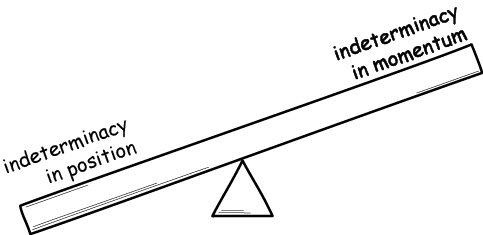...Applied to a Hydrogen Atom

 Since h is such a small number, the sorts of indeterminacies arising are so small as to be unnoticeable for ordinary objects. It is quite different on an atomic scale. Take the case of an electron trapped in a hydrogen atom. Let's think about it classically. If the electron is to remain bound to the positively charged nucleus of the atom, it must have a quite small momentum. Then it will remain in the familar elliptical orbit of Bohr's theory. (Or if we think fully classically, it will spiral into the nucleus as it radiates away its energy.)If the momentum is too big, the electron will tear itself away from the nucleus and escape. The electrical attraction of the nucleus will not be sufficient to hold it. This situation is essentially the same as what happens with a very rapidly moving comet and the sun. If the comet moves slowly enough, it will remain trapped in an elliptical orbit around the sun. If it is moving fast enough, it will flee off into space never to return. Now recall that these particles are matter waves subject to Heisenberg's principle. The indeterminacy in the momentum of the electron must be small. For only then are we assured that the momentum of the electron remains close enough to zero for it to remain trapped by the attraction of the nucleus. If the indeterminacy is large, we cannot preclude the possibility that the electron has a sufficiently large momentum to escape.

It is a simple computation to see how small that indeterminacy in the electron's momentum must be. If we then insert that smallest indeterminacy into Heisenberg's formula, we find the least indeterminacy of the electron's position. That indeterminacy in position turns out to be roughly of the size of the atom; or, more precisely, of the lowest energy orbit of Bohr's 1913 model.

 So the electron is spread over the whole atom; it is futile to look at a particular spot within the atom for the electron. This reflects what we already expected from the use of a matter wave to represent an electron in a hydrogen atom. Bohr's troublesome classical orbits are replaced by waves spread over the space surrounding the nucleus. These waves are often pictured as diffuse "clouds." The simplest of these clouds is pictured at right. Of course the nucleus is also subject to quantum mechanics, so it too should be "fuzzed out" into a little cloud.If we superimpose this electron cloud on the earlier picture of the Bohr atom with its definite electron orbits, the results looks like this:More generally, this idea of the electron cloud is the basis of the fact we saw in the last chapter, that electrons bound in an atom live in the orbitals that prove fundamental to modern chemistry. These are three dimensional analogs of the standing waves musicians find  vibrating on the one dimenionsal strings of their stringed instruments.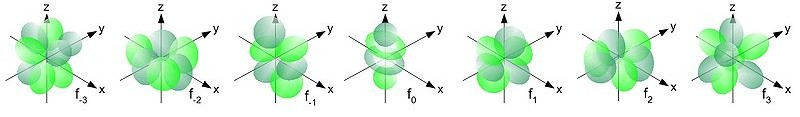http://commons.wikimedia.org/wiki/File:Single_electron_orbitals_s.jpg http://commons.wikimedia.org/wiki/File:Single_electron_orbitals_p1.jpg http://commons.wikimedia.org/wiki/File:Single_electron_orbitals_d.jpg http://commons.wikimedia.org/wiki/File:Single_electron_orbitals_f.jpg

Complementary Pairs

This reciprocal indeterminacy of position and momentum is just one of many in quantum mechanics. When two quantities form complementary pairs, the two quantities will enter into analogous indeterminacy relations. There is such a relation, for example, between the energy and timing of a process. There is another between the angular momentum of an object and its angular position.

 Angular momentum is the rotational analog of the ordinary "linear" momentum we investigated in an earlier chapter. Linear momentum, loosely speaking, is the physical quantity that measures the amount of motion of a moving body. This measure accommodates not just the speed but also the mass of the moving body. More massive bodies, moving at the same speed, carry more momentum. The amount of rotational motion of a rotating body, like a spinning top, is measured in a corresponding manner by its angular momentum. More massive bodies, spinning at the same speed, carry more angular momentum. Instead of mass, the quantity that contributes to angular momentum is the mass-derived "moment of inertia." Both linear and angular momentum have special places in physical theory since they are both conserved quantities.The other variable in the complementary pair is the angular position of the object. That is just a specification of the direction in which it lies with respect to some suitably chosen center and axis. Is it in the zero degree position? Or do we find it at 90 degrees? A familiar example of angular position is a compass bearing at sea. Our port, we might judge, lies due East, that is 90 degrees from due North. For the case of the top, we would find some mark on its rim. Its angular position would be the angular position of that mark around the rim, where we use the axis of rotation of the top as the axis around which the angles are measured.

This last indeterminacy can be applied to the example of the hydrogen atom. If an orbiting electron is definitely in just one of Bohr's stationary orbits, then its angular momentum has a definite value. It is one of these:

Angular Momentum
= one h/2π, two h/2π, three h/2π, . . .

As a result of the angular momentum-angular position indeterminacy, its angular position must be completely indeterminate. So the angular position of the electron about an axis used to determine the angular momentum is completely indeterminate. That is again just what we would expect when we replace Bohr's point-like electrons with waves.

Uncertain or Indefinite?

Why am I avoiding the common talk of "uncertainty" in association with Heisenberg's principle?

 Uncertainty over some quantity suggests the quantity has a definite value but that we just do not know what it is. We may be uncertain, for example, about the price of a paint set at the art store before we go there to buy it. There is a definite price all customers are charged; we just do not know what it is.http://www.wpclipart.com/art/paints/watercolors_paint_tray.png.html For sale: \$5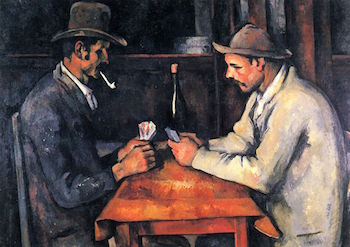For sale: highest bidder Now compare that with the price that some very valuable painting may obtain in a coming auction. We do not now know what that price will be; the auction hasn't happened yet. We may say that we are uncertain of the price. But it is a different sort of uncertainty. There is no price now to know. The price will only be determined when the auction actually happens.

In the standard approach to quantum mechanics, the uncertainties of Heisenberg's uncertainty principle are of the second type. When the position of a particle is indeterminate, that means that there is no single position associated with the particle; its wave is spread over many positions. It is not that the particle really has a definite position and we just don't know which it is. It is not that we are uncertain about the position because there are more facts to know about the position. There are no further facts to know.

So talk of "uncertainty" in Heisenberg's formula can be misleading. It suggest that we are just ignorant of something that could be known. It is easy to overlook the second way that we can come to be uncertain: the issue is indefinite and there is nothing more to know.

The standard approach to quantum mechanics derives the uncertainty from indefiniteness. There are other approaches in which this is not so. In one developed by Louis de Broglie and David Bohm, particles always have a definite position and the uncertainties arise from our ignorance. These approaches represent a minority view.

How Quantum States Change over Time

Schroedinger Evolution...

An essential part of quantum mechanics deals with how matter waves change over time. Mostly, matter waves behave just like ordinary waves. If you have ever watched ripples spread on the surface of a smooth pond, you have see at least qualitatively just what matter waves do.Take a particle that we localize to just one place, so its matter wave is a spatially localized pulse. Left to itself, that pulse will spread out in all directions as propagating waves. It is just like what happens when a pebble hits the surface of the pond. The localized splash immediately spreads out in broadening ripples.That type of behavior is called "Schroedinger evolution," because it is governed by Schroedinger's wave equation.That equation just says that matter waves propagate like waves.

...Is Not the Whole Story

If Schroedinger evolution were the only way that matter waves could change, we would have some difficulty connecting matter waves with our ordinary experience. Matter waves typically are spread over many positions and are superpositions of many momenta. Yet when we measure them, we always find just one value for position or momentum.

For example, the simplest sort of measurement is to intercept a matter wave with a photographic plate or a scintillation screen that glows when struck by a particle. In both cases, we find that the matter waves yield just one definite position. They give us a single spot in the photograph or a localized flash of light on the screen.

 The screen of an old fashioned TV tube is a scintillation screen. Electrons are fired at it from an electron gun at the rear of the tube. While the electrons are in flight, they retain wavelike properties. Those wavelike properties are essential to an electron microscope, which focusses them like an optical microscope focusses light.When the matter wave of the electron strikes the screen, however, the resulting flash of light reveals just a single position.

Measurement: Collapse of the Wave Packet

The standard solution to this problem is to propose that there is a second sort of time evolution for matter waves. The first type, Schroedinger evolution, arises when matter waves are left to themselves or when they interact with just a few other particles.

The second type arises whenever we perform a measurement of a quantity like position or momentum. Then the matter wave collapses to one that has a definite value for the quantity measured. If we are measuring the position of the matter wave, it collapses to a localized pulse. If we are measuring momentum, it collapses to a wave with a definite momentum.

This second sort of time evolution is called "measurement" or "collapse of the wave packet."It is not easy to specify exactly when a measurement evolution will take place. The simplest condition is that it arises in a circumstance in which we are trying to ascertain the value of a quantity. That condition is of no use in theory formation. For matter waves do not "know" what we are intending; they do not choose to evolve in one way or another according to our wishes or interests. The best we can come up with is a simple rule of thumb. Matter waves left to themselves or interacting with just a few particles undergo Schroedinger evolution. Matter waves interacting with macroscopic bodies (such as particle detectors) undergo collapse.

Indeterminism: An Unsure Future

Schroedinger evolution of a matter wave is fully deterministic. That means that if we specify the present state of the matter wave, its future state is fixed completely by Schroedinger's equation.

This determinism of the theory fails when we consider measurement. For when we measure the position of a particle represented by a wave packet, we do not know for sure which position will be revealed. The best we can do is to say which are the candidate positions and, using a standard rule, compute the probability of each.

Thus measurement introduces indeterminism into quantum theory. A full specification of the present state of the matter wave and everything that will interact with it is not enough to fix what its future state will be.The rule that determines the probability of each candidate outcome depends essentially on superposition. Consider, for example, a wave packet. It is the superposition of many spatially localized pulses. The figure shows just five of them. In general there are infinitely many. What is important is that the amplitude of the component pulses vary according to the part to which they will contribute in the fully assembled wave packet. A pulse contributing to the large amplitude central section will have a large amplitude. A pulse contributing to the smaller amplitude edges will itself have a smaller amplitude. This last fact is the clue that tells us how to compute the probability of a measurement outcome. We expect the measured position of the particle to appear more probably in the large amplitude center of the wave packet, than in the lower amplitude edges.

Max Born used this fact when he proposed the "Born rule," that tells us that the amplitude of the component fixes the probability that this component will be the outcome of measurement.

 Probability that wave packet collapses to component on measurement = ( amplitude of component ) 2

 The slight complication in Born's rule is that the amplitudes of the components are not real numbers. They are complex numbers that include things like "i," the square root of minus one and other more complicated things like 1+i and 37 - 10i. Probabilities have to be real numbers between 0 and 1. So Born had to convert the complex-valued amplitudes into a real numbers. There are many ways of doing this. Few give a real number that also obeys all the rules of the probability calculus. Taking the "square" of the amplitude turns out to be the one that works. For more, see A Complex Wave. For experts only: of course by "square" of a complex number I really mean its "squared norm." That is the number itself, multiplied by its complex conjugate. For z=1+i, the squared norm|z|2 = (1+i)(1-i) = 1-i2 = 2.

Anxieties over Irreducible Chanciness

When quantum theory first emerged as our best theory of fundamental particles, the central role of probabilities in the theory caused much concern. The probabilities associated with the collapse of the wave packet were not of the type always formerly seen.

Prior to quantum theory, the probabilities that had crept into physics could always be thought of as manifestations of our ignorance of the true state of affairs.

We might not know whether a coin will come up heads or tails when tossed, so we say there is a probability of 1/2 on heads. But that probability merely masks our ignorance. If we knew exactly how hard the coin had been flipped, exactly how the air currents in the room were laid out, and a myriad more other details, we could in principle determine exactly whether the coin would be heads or tails.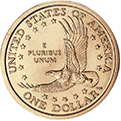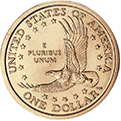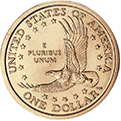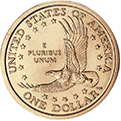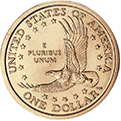In quantum theory, when the wave packet collapses, we find different probabilities for the different outcomes. But there is no definite fact of the matter over which we are ignorant. There is no one true, hidden outcome prior to measurement. No further accumulation of information could lessen our ignorance. There is nothing more to know. The best we can say is that each of the position measurements are possible and that they will arise with such and such probability.

It is now a little hard to see why this difference in the probabilities led to so much anxiety among physicists in the 1920s and later. All that has happened is that we have found the world to be a little different from what we expected. We may once have thought probabilities to be expressions of ignorance. We now find that they are irreducible parts of the way the world is put together. Their appearance in theory has nothing to do with what we may or may not know. The world just is fundamentally chancy in certain of its aspects.

The Nineteenth Century View of Causation

The reason, I believe, that this irreducibly chancy character of the world created such anxiety is a legacy of nineteenth century philosophy. In the course of the nineteenth century, the notion of causation had been greatly purified by philosophical analysis. The outcome was a lean account of causation as determinism. This causes that simply means that this is invariably followed by that. So for the world to be causal, in this view, simply means that the present state of the world fixes its future state.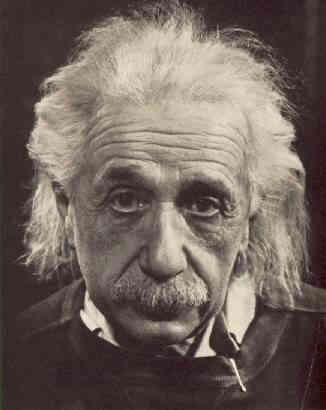It may now be hard to see that this is what the nineteenth century scientists took causality to be. Here is Einstein, in a speech from 1950, describing the situation: "...the laws of the external world were also taken to be complete, in the following sense: If the state of the objects is completely given at a certain time, then their state at any other time is completely determined by the laws of nature. This is just what we mean when we speak of 'causality.' Such was approximately the framework of the physical thinking a hundred years ago." Albert Einstein, "Physics, Philosophy, and Scientific Progress," International Congress of Surgeons, Cleveland, Ohio, 1950; printed in Physics Today, June 2005, pp.46-48.

The irreducible probabilities of quantum theory showed that the present state of the world does not fix its future state. The best it does is to give probabilities for different possible futures. Therefore, according to the nineteenth century conception, the world is not causal. Thus the physicists of the 1920s frequently lamented the violation of the "principle of causality."

 The consensus now is that their notion of causation was far too narrow. There are notions of causation that cohere perfectly well with irreducible probabilities. Quantum theory does not present a challenge to the cogency of causation. We now think that quantum mechanics does not present a foundational problem in this area. However quantum theory does present some significant foundational problems in related areas. These problems will be the subject of the following chapters. That is the majority view. There is a minority view, which I champion. It regards the 1920s failure of the principle of causality as part of a long history of failure. In this view, the effort to find a principle of causality in nature is actually an effort to conceive an a priori science. Processes in nature are interconnected. But it is not our business to legislate in advance the nature of that connectedness. Perhaps it conforms to something like a principle of causality; or perhaps it does not. The long history of our failure to find any well-functioning principle of causality suggests that there is none to be found. It suggests that our efforts are better spent empirically examining how things connect, broadening our conceptions to match and not trying to force them into a mold first devised thousands of years ago. Or that is what I argue in my "Causation as Folk Science." in Philosophers' Imprint, Vol. 3, No. 4.

What you should know

• How matter waves enter into superpositions and how this allows wave packets to form.
• How Heisenberg's uncertainly principle places a limit of the definiteness of quantities.
• The difference between uncertainty and indefiniteness.
• What is quantum measurement (collapse of the wave packet).
• How probabilities essentially enter into quantum theory and why this was initially regarded as a failure of causality.

Copyright John D. Norton. April 2001; March 16, Augst 22, December 1, 2008; March 5, April 14, 2010; April 7, 2015. April 14, 2017.